Ex 5.2

Chapter 5 Class 11 Complex Numbers (Term 1)
Serial order wise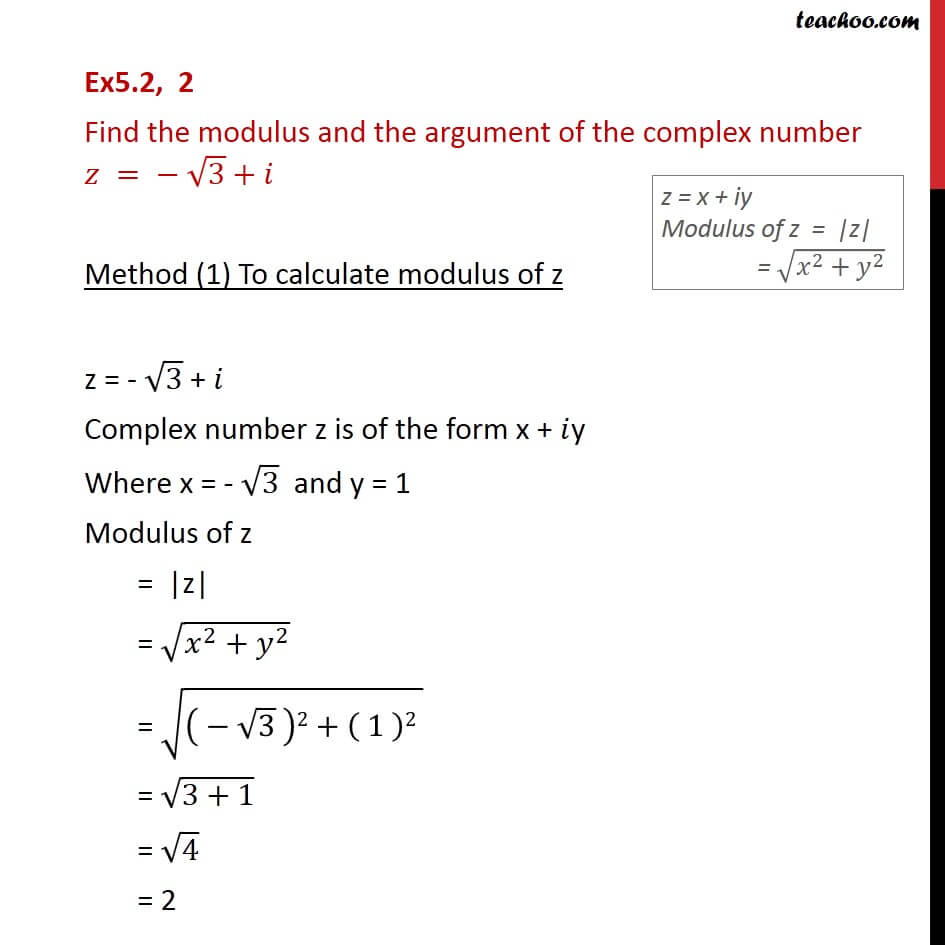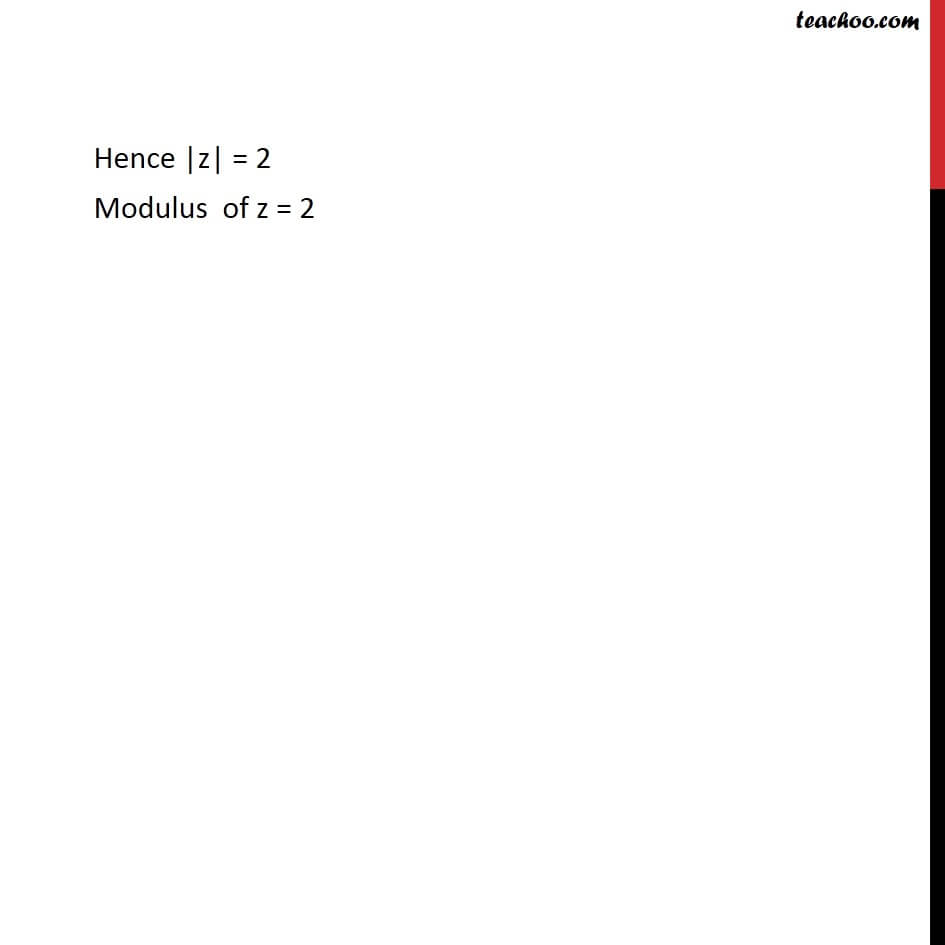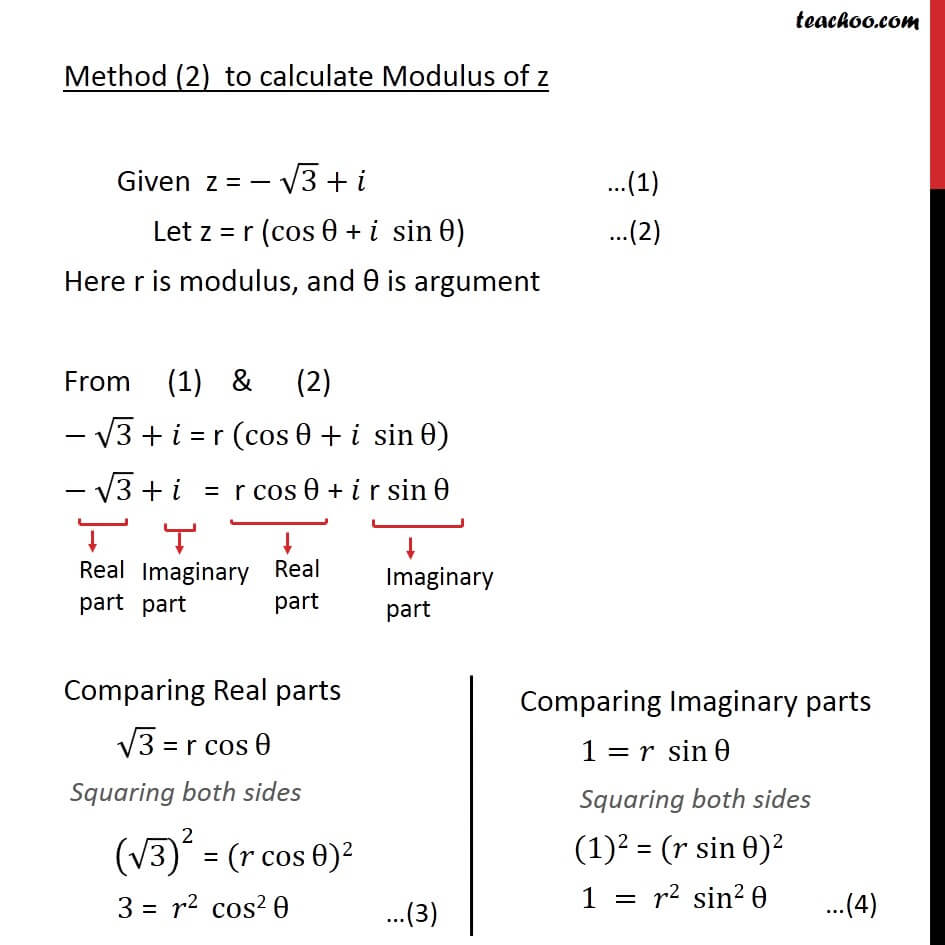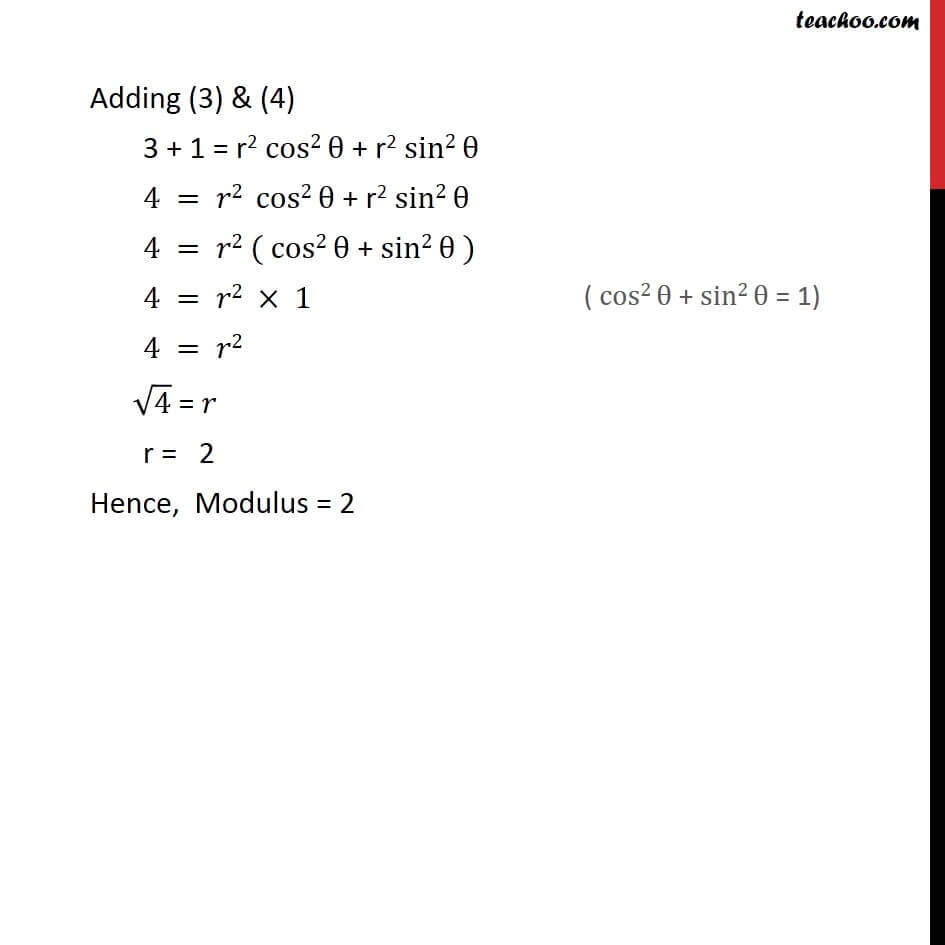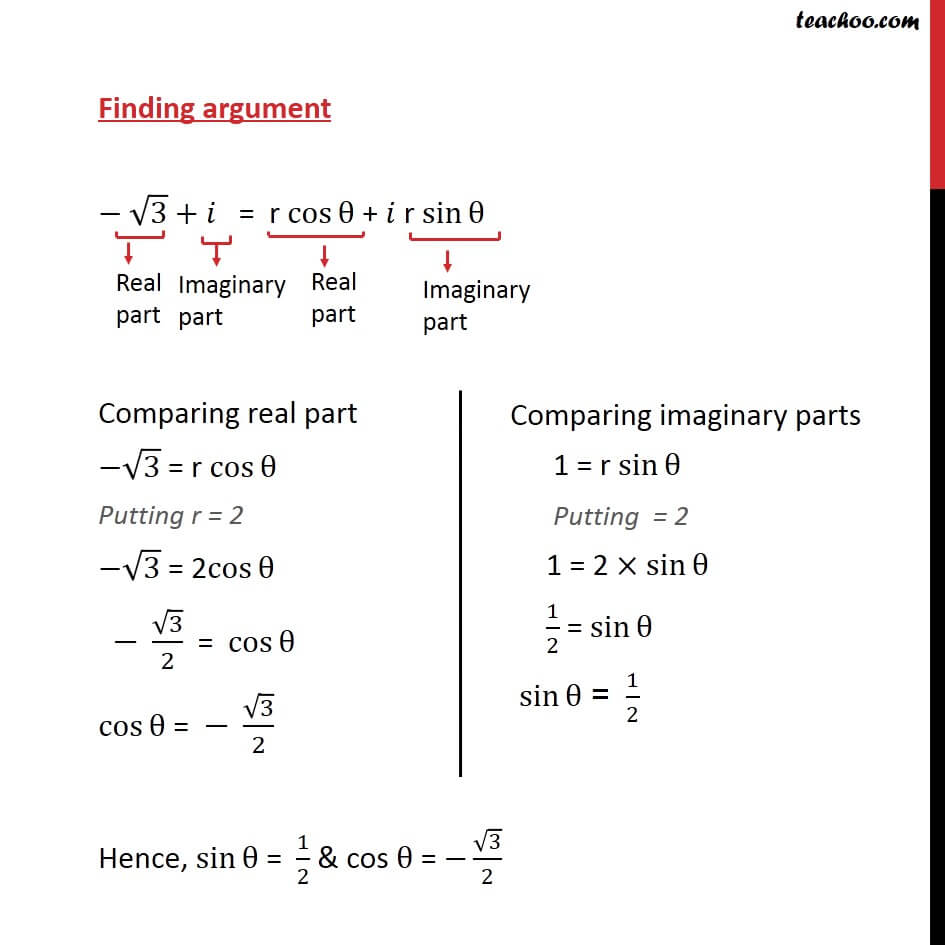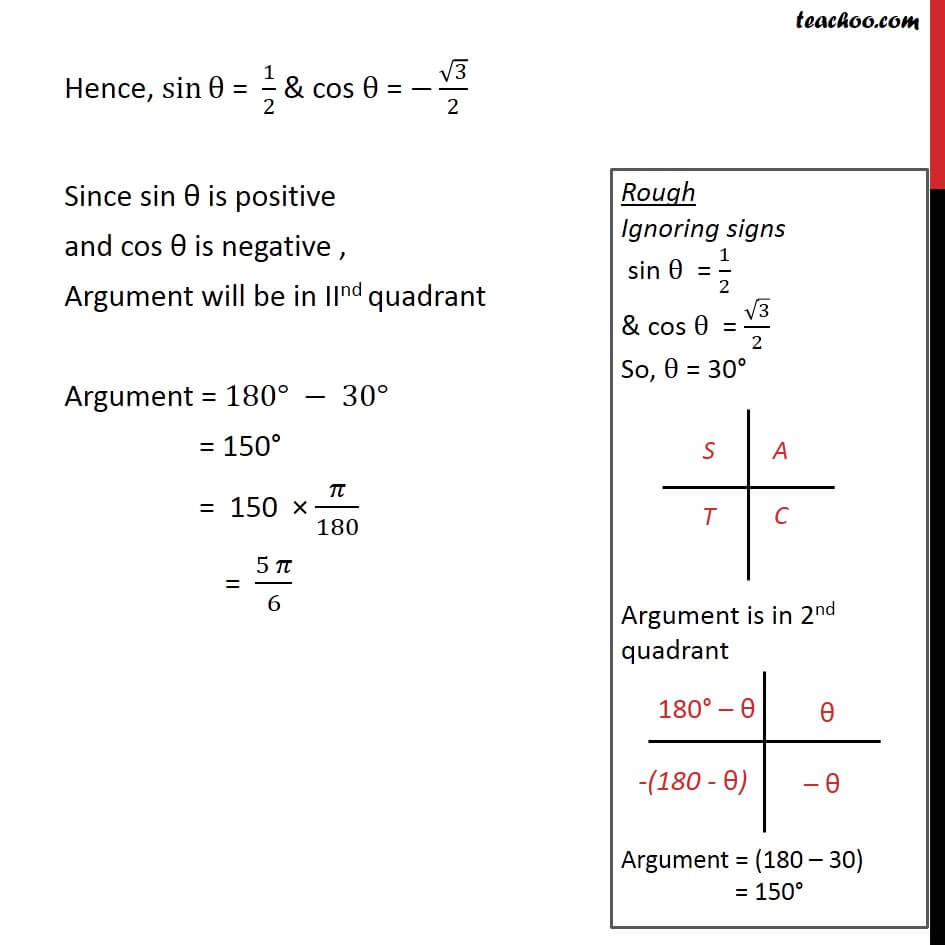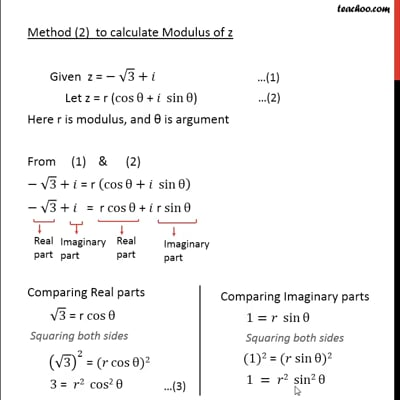This video is only available for Teachoo black users

### Transcript

Ex5.2, 2 Find the modulus and the argument of the complex number 𝑧 = − √3 + 𝑖 Method (1) To calculate modulus of z z = - √3 + 𝑖 Complex number z is of the form x + 𝑖y Where x = - √3 and y = 1 Modulus of z = |z| = √(𝑥^2+𝑦^2 ) = √(( − √3 )2+( 1 )2 ) = √(3+1) = √4 = 2 Hence |z| = 2 Modulus of z = 2 Method (2) to calculate Modulus of z Given z = − √3 + 𝑖 Let z = r (cos⁡θ + 𝑖 sin⁡θ) Here r is modulus, and θ is argument From (1) & (2) − √3 + 𝑖 = r (cos⁡θ+𝑖 sin⁡θ ) − √3 + 𝑖 = r〖 cos〗⁡θ + 𝑖 r sin⁡θ Comparing Real parts √3 = r cos⁡θ Squaring both sides (√3)^2 = (𝑟 cos⁡θ)2 3 = 𝑟2 〖 cos"2" 〗⁡θ Adding (3) & (4) 3 + 1 = r2 cos2⁡θ + r2 sin2⁡θ 4 = 𝑟2 cos2⁡θ + r2 sin2⁡θ 4 = 𝑟2 ( cos2⁡θ + sin2⁡θ ) 4 = 𝑟2 × 1 4 = 𝑟2 √4 = 𝑟 r = 2 Hence, Modulus = 2 Finding argument − √3 + 𝑖 = r〖 cos〗⁡θ + 𝑖 r sin⁡θ Comparing real part −√3 = r cos⁡θ Putting r = 2 −√3 = 2cos⁡θ − √3/2 = cos⁡θ cos⁡θ = − √3/2 Hence, sin⁡θ = 1/2 & cos θ = −√3/2 Since sin θ is positive and cos θ is negative , Argument will be in IInd quadrant Argument = 180° − 30° = 150° = 150 × 𝜋/180 = (5 𝜋)/3